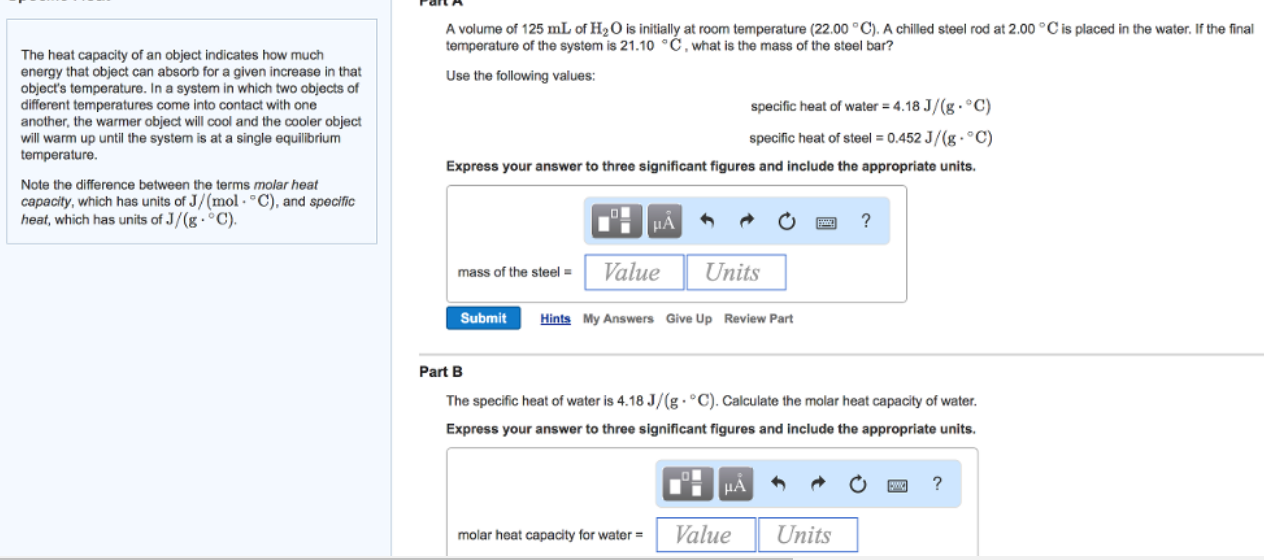# Problem: The heat capacity of an object indicates how much energy that object can absorb for a given increase in that Use the following values: object's temperature. In a system in which two objects of specific heat of water 4.18 J/(g.°C) different temperatures come into contact with one another, the warmer object will cool and the cooler object specific heat of steel 0.452 J/(g C) will warm up until the system is at a single equilibrium temperature.Note the difference between the terms molar heat capacity, which has units of J/(mo C), and specific heat, which has units of J/(g. C).A volume of 125 mL of H2O is initially at room temperature (22.00 C), A chilled steel rod at 2.00 C is placed in the water. If the final temperature of the system is 21.10C, what is the mass of the steel bar?The specific heat of water is 4.18 J/(g. C). Calculate the molar heat capacity of water.Express your answer to three significant figures and include the appropriate units.°

###### FREE Expert Solution
81% (220 ratings)###### Problem Details

The heat capacity of an object indicates how much energy that object can absorb for a given increase in that Use the following values: object's temperature. In a system in which two objects of specific heat of water 4.18 J/(g.°C) different temperatures come into contact with one another, the warmer object will cool and the cooler object specific heat of steel 0.452 J/(g C) will warm up until the system is at a single equilibrium temperature.

Note the difference between the terms molar heat capacity, which has units of J/(mo C), and specific heat, which has units of J/(g. C).
A volume of 125 mL of H2O is initially at room temperature (22.00 C), A chilled steel rod at 2.00 C is placed in the water. If the final temperature of the system is 21.10C, what is the mass of the steel bar?

The specific heat of water is 4.18 J/(g. C). Calculate the molar heat capacity of water.

Express your answer to three significant figures and include the appropriate units.°

Frequently Asked Questions

What scientific concept do you need to know in order to solve this problem?

Our tutors have indicated that to solve this problem you will need to apply the Thermal Equilibrium concept. You can view video lessons to learn Thermal Equilibrium. Or if you need more Thermal Equilibrium practice, you can also practice Thermal Equilibrium practice problems.

What professor is this problem relevant for?

Based on our data, we think this problem is relevant for Professor King & Ilies' class at DREXEL.